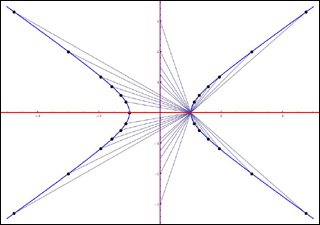18.781 | Spring 2012 | Undergraduate

# Theory of Numbers

#### Course Description

This course is an elementary introduction to number theory with no algebraic prerequisites. Topics covered include primes, congruences, quadratic reciprocity, diophantine equations, irrational numbers, continued fractions, and partitions.

## Course Info

##### Learning Resource Types
Problem Sets with Solutions
Exams with Solutions
Lecture NotesSome rational points on the hyperbola x^2 - 2y^2 = 1. The projection from away from (1,0) gives a bijection with the rational points on the y-axis, with the point (0,-m) going to x = (2m^2 + 1)/(2m^2 - 1), y = 2m/(2m^2 - 1). (Image by Abhinav Kumar.)